flat assembler
Message board for the users of flat assembler.Index > Macroinstructions > 8) Square root macro for numerical constants
Author
 ThreadMCD

Joined: 21 Aug 2004
Posts: 604
Location: Germany
MCD
Here is another brainchild of mine!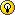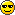I have a nice square root macro that works on Flat Assembler integer
numerical constants at COMPILE TIME :]. (For beginners: if you want to
calculate square roots at runtime, use something like "fsqrt" instruction.)

It uses a sophisticated Heron method, thus only 7 iterative steps are
required in a worst case scenario to give the full 63bit Fasm precision.
Note that this macro works only on integer values (see Fasm documentation),
thus the returned square roots are rounded.
Furthermore and unfortunately, Fasm allows only complicated and slow early
out loops because the "repeat"-directive doesn't allow quit conditions, but
I think that this won't hurt too much.

Here is an usage example:
Code:
```;The DispDec macro displays numerical constants in decimal
macro   DispDec [Val]
{
if Val <> 0
Acc= Val
Divider= 1000000000000000000
Highest0= 0
if Acc < 0
display "-"
end if
repeat 19
Digit= Acc/Divider
Acc= Acc mod Divider
Divider= Divider/10
if Digit <> 0
Highest0= 1
end if
if Highest0 = 1
display Digit+"0"
end if
end repeat
else
display "0"
end if
}

SomeVar= 6432168469798324165;Any positive value up to 2^63 - 1 goes
SqRt SomeVar;This is where the magic happens!
DispDec SomeVar
```

Watch out: unfortunately SqRt cannot take immediates! so this code generates
an error:
Code:
`SqRt 98654    `

Last edited by MCD on 06 Jan 2005, 19:09; edited 3 times in total05 Jan 2005, 20:30MCD

Joined: 21 Aug 2004
Posts: 604
Location: Germany
MCD
Here goes the actual SqRt macro:
Code:
```macro SqRt    Val
{
if Val <> 0
if Val >= 0;These lines serve to guess a good and fast starting value
Acc= 11
if Val >= 100h
Acc= 171
if Val >= 10000h
Acc= 2740
if Val >= 1000000h
Acc= 43838
if Val >= 100000000h
Acc= 701416
if Val >= 10000000000h
Acc= 11222655
if Val >= 1000000000000h
Acc= 179562481
if Val >= 100000000000000h
Acc= 2038618457
end if
end if
end if
end if
end if
end if
end if
Val= Val-1;This is just a rounding stuff
repeat 7
Acc= (Val/Acc + Acc +1) shr 1
end repeat
Val= Acc
else
Acc= 1/0;This will intentionally cause an "value out of range" error!
end if
end if
}
```

_________________
MCD - the inevitable return of the Mad Computer Doggy

-||__/
.|+-~
.|| ||05 Jan 2005, 20:31MCD

Joined: 21 Aug 2004
Posts: 604
Location: Germany
MCD
If you need a faster SqRt macro and you can sacrify some precision, then you
could use less iterative starting value steps or unroll the loop a bit
(not sure if this speeds it up?) or decrease the repeat number below 7. But
in any case, more than 7 repeats only slow it down.

The starting value stuff is difficult to optimize. If you use less steps, you
will have to use more repeats. Thus, both too much/less steps slow down the
iterative process. I tried out many different step numbers from 1 to 16, and
8 seem to be the gold middle. I tested it with the following code (this may
take some time on older machines):
Code:
```repeat 40000h
X= % - 1
SqRt X
end repeat

repeat 40000h
X= % shl 45 - 1
SqRt X
end repeat
```

Does anyone know a faster square root algorythm than Heron(except the Taylor
based stuff)? I would also know a way to optimize the starting value guess by
using a "bsr" operator, but I don't think it is worth beeing implemented. It
would give something like...
Code:
```;...
Acc= 1 shl (bsr Val shr 1);Small, but very effective;...
```

...instead of all these ugly 8 nested if-blocks.

_________________
MCD - the inevitable return of the Mad Computer Doggy

-||__/
.|+-~
.|| ||05 Jan 2005, 20:32MCD

Joined: 21 Aug 2004
Posts: 604
Location: Germany
MCD
Any need for number displaying macros? Try these:
Code:
```macro     DispDec [Val]
{
if Val <> 0
Acc=Val
Divider=1000000000000000000
Highest0=0
if Acc < 0
display "-"
end if
repeat 19
Digit= Acc/Divider
Acc= Acc mod Divider
Divider= Divider/10
if Digit <> 0
Highest0= 1
end if
if Highest0 = 1
display Digit+"0"
end if
end repeat
else
display "0"
end if
}

macro       DispHex [Val]
{
if Val <> 0
Acc=Val
Divider=60
Highest0=0
repeat 16
Digit= (Acc shr Divider) and 0Fh
Acc= Acc and (1 shl Divider - 1)
Divider= Divider-4
if Digit <> 0
Highest0= 1
end if
if Highest0 = 1
if Digit < 0Ah
display Digit+"0"
else
display Digit+"A"-0Ah
end if
end if
end repeat
else
display "0"
end if
}

macro      DispBin [Val]
{
if Val <> 0
Acc=Val
Divider=63
Highest0=0
repeat 64
Digit= (Acc shr Divider) and 1
Acc= Acc and (1 shl Divider - 1)
Divider= Divider-1
if Digit = 0
if Highest0 = 1
display "0"
end if
else
display "1"
Highest0=1
end if
end repeat
else
display "0"
end if
}
```05 Jan 2005, 20:32beppe85

Joined: 23 Oct 2004
Posts: 181
beppe85
Cool!

Why you not put two versions, with an equate naming with routine to use The dev usually will use the precise operation only on final builds.05 Jan 2005, 20:38MCD

Joined: 21 Aug 2004
Posts: 604
Location: Germany
MCD
Quote:
Why you not put two versions, with an equate naming with routine to use The dev usually will use the precise operation only on final builds.
... hdsfjka osÃ¶fsdghi sfgisdgsd f24h3dg hs4df,w3215476095(/&\$Â§)(/Q/%\$\$%Â§"\$EW i jdoigds???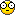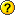I don't understand a word, please try to form a correct sentence.05 Jan 2005, 20:58MCD

Joined: 21 Aug 2004
Posts: 604
Location: Germany
MCD
Anyone else intereted in this SqRt macro?06 Jan 2005, 19:06beppe85

Joined: 23 Oct 2004
Posts: 181
beppe85
The day you form a correct and useful array of assembly instructions, I'll learn a foreign language.

MCD wrote:
Quote:
Why you not put two versions, with an equate naming with routine to use The dev usually will use the precise operation only on final builds.
... hdsfjka osÃ¶fsdghi sfgisdgsd f24h3dg hs4df,w3215476095(/&\$Â§)(/Q/%\$\$%Â§"\$EW i jdoigds???I don't understand a word, please try to form a correct sentence.10 Jan 2005, 00:09Pharabee Joined: 18 Nov 2003 Posts: 16 Location: Sukabumi,Indonesia Pharabee Im still confused with square root. Fsqrt take 100 ms if I dont made mistake on 10 000 000 loop. _________________How to Get work Fast: 10% Skill, 90% honesty.27 Jul 2005, 09:01
MCD

Joined: 21 Aug 2004
Posts: 604
Location: Germany
MCD
As I said already (somewhere even I don't remind where), this macro works at compile-time, whereas fsqrt should be used for runtime square root calculation. Do you know what the difference between compile and runtime calculations is?27 Jul 2005, 14:33Pharabee Joined: 18 Nov 2003 Posts: 16 Location: Sukabumi,Indonesia Pharabee No I dont know the different. _________________How to Get work Fast: 10% Skill, 90% honesty.27 Jul 2005, 15:50
 revolution When all else fails, read the source Joined: 24 Aug 2004 Posts: 17484 Location: In your JS exploiting you and your system revolution Here is my try that avoids the chain of IF's Code:```macro two_pow_half_log2 Val { Acc=Val Acc=Acc or (Acc shr 1) Acc=Acc or (Acc shr 2) Acc=Acc or (Acc shr 4) Acc=Acc or (Acc shr 8) Acc=Acc or (Acc shr 16) Acc=Acc or (Acc shr 32) Acc=Acc-((Acc shr 1) and 05555555555555555h) Acc=(((Acc shr 2) and 03333333333333333h)+(Acc and 03333333333333333h)) Acc=(((Acc shr 4)+Acc) and 00f0f0f0f0f0f0f0fh) Acc=Acc+(Acc shr 8) Acc=Acc+(Acc shr 16) Acc=((Acc+(Acc shr 32)) and 07fh)-1 Acc=1 shl (Acc shr 1) } macro SqRt In { local Val Val=In if Val>0 two_pow_half_log2 Val Val=Val-1 repeat 7 Acc=(Val/Acc+Acc+1) shr 1 end repeat else Acc=0 end if } macro display_decimal value { local leading_zero,digit,temp,number,divisor number=value if number=1 shl 63 display '-9223372036854775808' else if number<0 number=-number display '-' end if leading_zero=0 divisor=1000000000000000000 repeat 19 digit=19-% temp=(number/divisor) mod 10 divisor=divisor/10 leading_zero=leading_zero+temp if leading_zero | (digit=0) display temp+'0' end if end repeat end if } macro show_SqRt Val { display_decimal Val display ' --> ' SqRt Val display_decimal Acc display 13,10 } ;some simple range testing rept 64 count:0 {show_SqRt (1 shl count)-1} display 13,10 rept 63 count:0 {show_SqRt 1 shl count} display 13,10 rept 63 count:0 {show_SqRt (1 shl count)+1} display 13,10 ;show the transition points rept 32 count:0 { show_SqRt ((count*10+5)*(count*10+5)/100) show_SqRt ((count*10+5)*(count*10+5)/100+1) } ``` But I don't know if the loop counter should 7 or 8 or more/less? My first approxmation is different than MCD gives.28 Jul 2005, 04:11
 Pharabee Joined: 18 Nov 2003 Posts: 16 Location: Sukabumi,Indonesia Pharabee Sorry I made mistake, fsqrt is 4905 ms in 10 mega loop. I think I will made a lookup table for this. I saw menuet OS 3D engine is very fast, maybe there are some info about this. What about that sqrt function speed? Did someone test it? _________________How to Get work Fast: 10% Skill, 90% honesty.28 Jul 2005, 04:52
Pharabee

Joined: 18 Nov 2003
Posts: 16
Location: Sukabumi,Indonesia
Pharabee
Here is my math function coded at MASM, use it for commercial or non commercial purpose.
Before GetSin and GetCos can be use you must call the Phase1 function first.
It will be great if we can colaboration on this math function. FASM is have a great documentation, average all the user is a good coder.

 Description: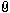Download Filename: fMath.inc Filesize: 11.96 KB Downloaded: 188 Time(s)

_________________
How to Get work Fast:
10% Skill, 90% honesty.28 Jul 2005, 04:57
MCD

Joined: 21 Aug 2004
Posts: 604
Location: Germany
MCD
Pharabee, maybe you should have a closer look into the fasm's programming manuel to understand the difference between runtime instructions and compile time macros.28 Jul 2005, 14:14Pharabee Joined: 18 Nov 2003 Posts: 16 Location: Sukabumi,Indonesia Pharabee I have understand, function use call and ret instruction thats why macro is faster. My sqrt look up table took 10ms on 10 M loop for gain the sqrt value, it mean 490 times faster than FPU. Im not too understand creating macro. _________________How to Get work Fast: 10% Skill, 90% honesty.30 Jul 2005, 06:12
 Display posts from previous: All Posts1 Day7 Days2 Weeks1 Month3 Months6 Months1 Year Oldest FirstNewest FirstJump to: Select a forum Official----------------AssemblyPeripheria General----------------MainDOSWindowsLinuxUnixMenuetOS Specific----------------MacroinstructionsCompiler InternalsIDE DevelopmentOS ConstructionNon-x86 architecturesHigh Level LanguagesProgramming Language DesignProjects and IdeasExamples and Tutorials Other----------------FeedbackHeapTest Area

Forum Rules:
 You cannot post new topics in this forumYou cannot reply to topics in this forumYou cannot edit your posts in this forumYou cannot delete your posts in this forumYou cannot vote in polls in this forumYou cannot attach files in this forumYou can download files in this forum